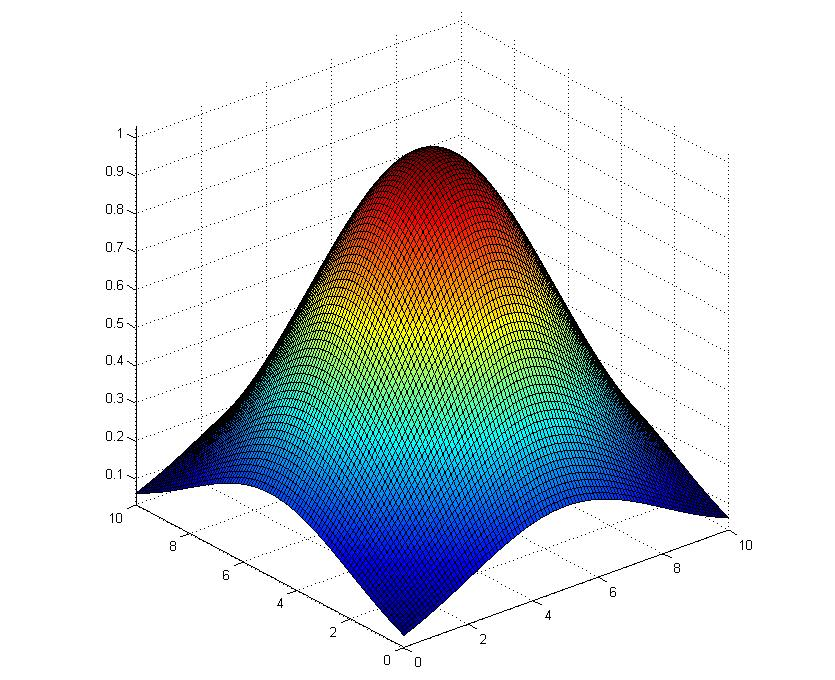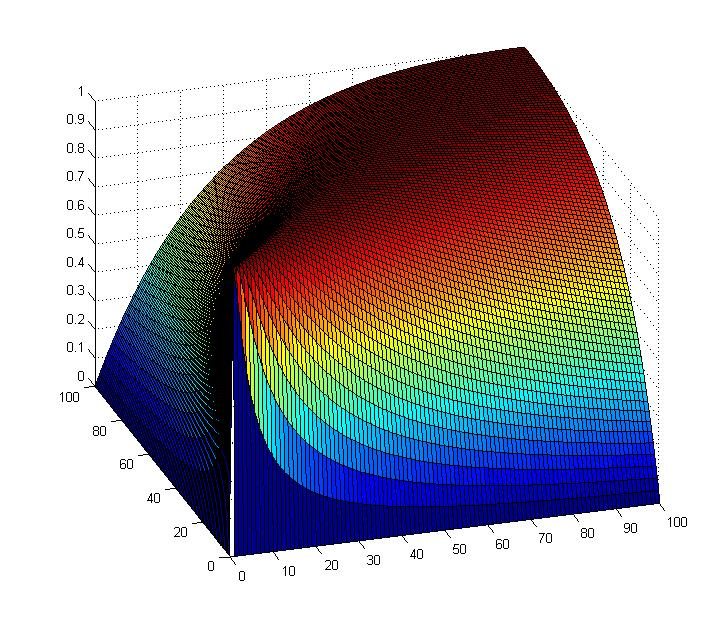# The Fourth Dimension Space

## 2-dimensional gauss function with Matlab (使用Matlab绘制二维高斯函数图象)

by using following codes.
%%
X1 = [0:0.1:10];
X2 = [0:0.1:10];
[X,Y] = meshgrid(X1,X2);
Z = exp(-((X-5).*(X-5)+(Y-5).*(Y-5))/18);
surf(X,Y,Z);
%%
the result is as following...2D gauss function.
and the distribution of 4*(x*y)/pow((x+y),2) is
X1 = [0:1:100];
X2 = [0:1:100];
[X,Y] = meshgrid(X1,X2);
Z = 4*X.*Y./(X+Y)./(X+Y);
surf(X,Y,Z);the relationship between eigenvalues of Harris Matrix

so we can see along the line y=x the value is maximized.

posted on 2012-11-14 21:27 abilitytao 阅读(3940) 评论(2)  编辑 收藏 引用@qiang